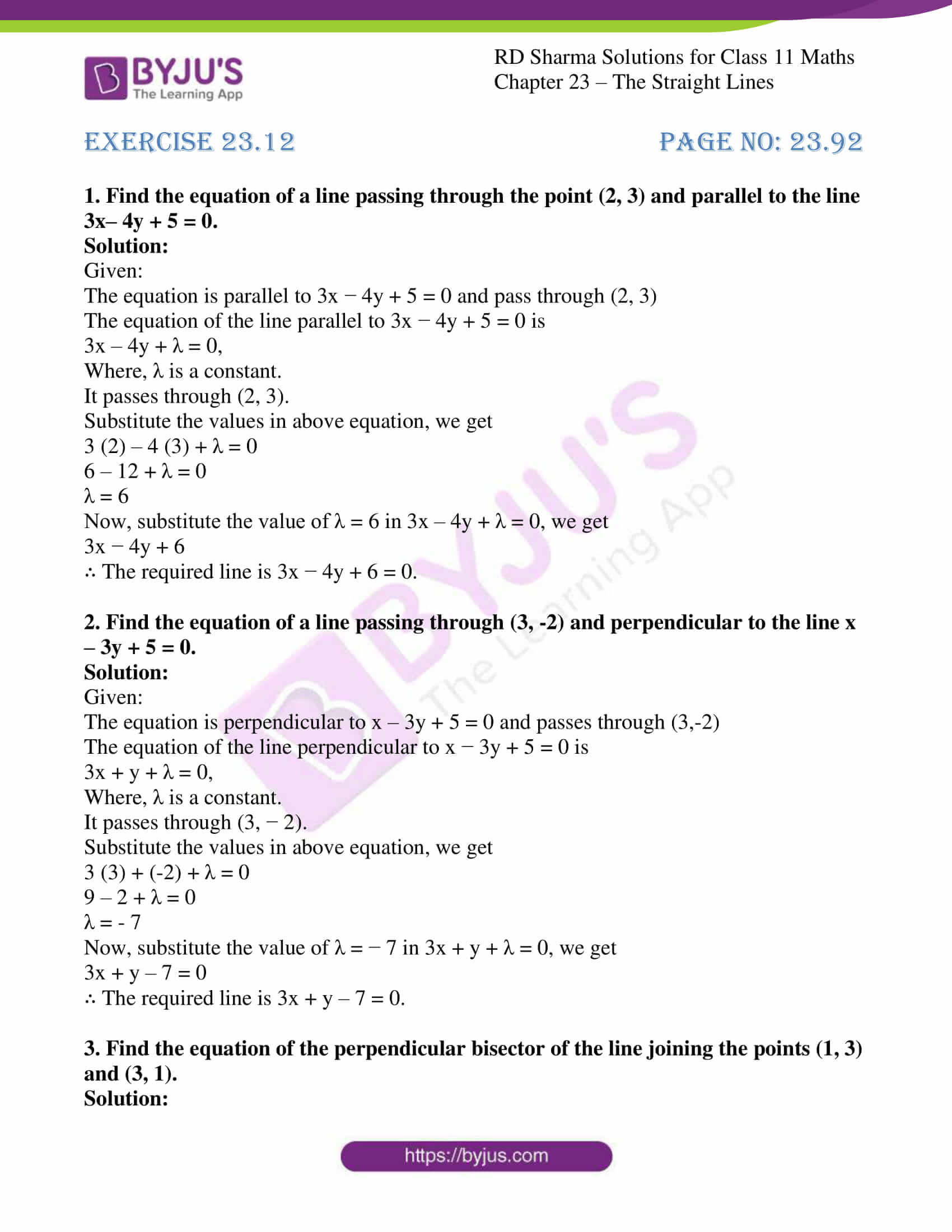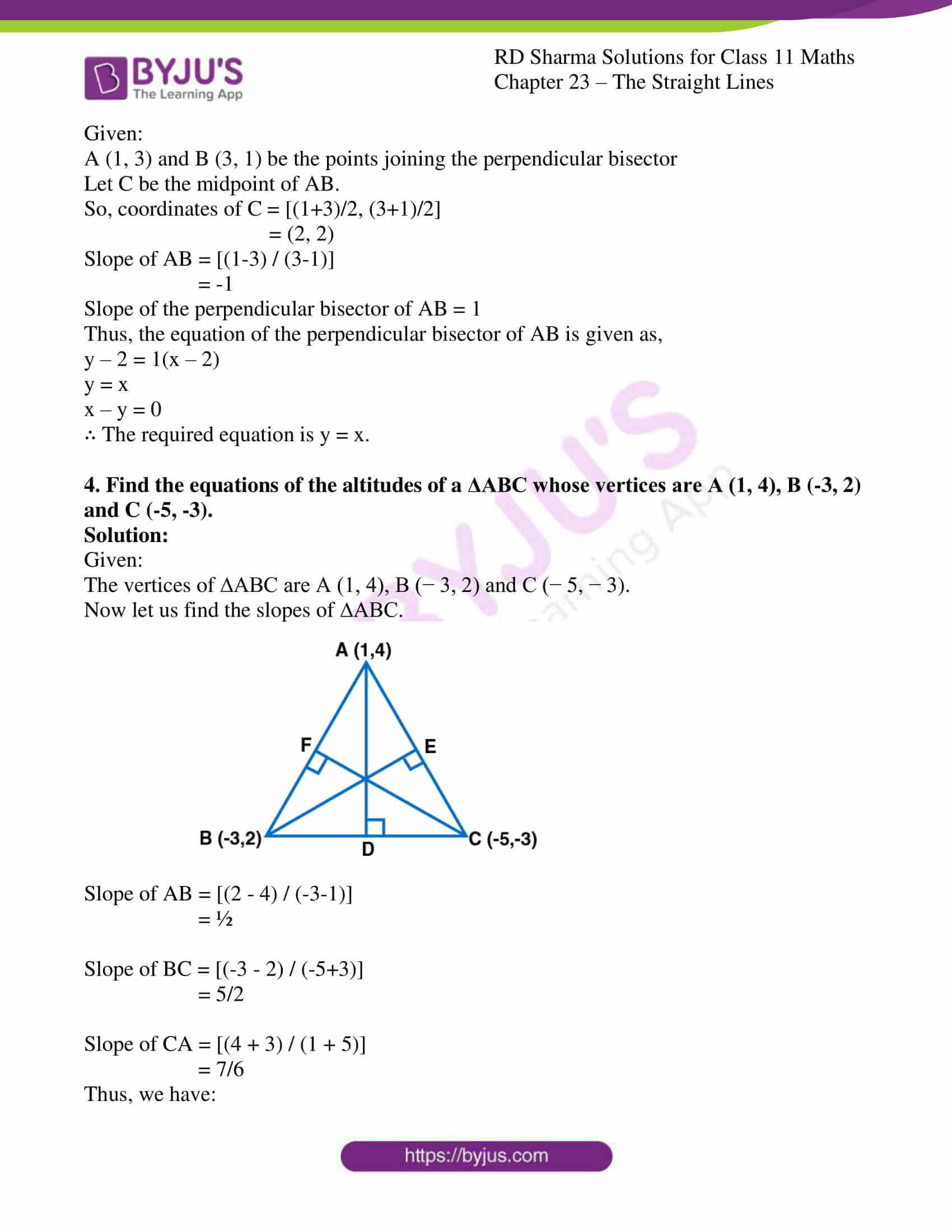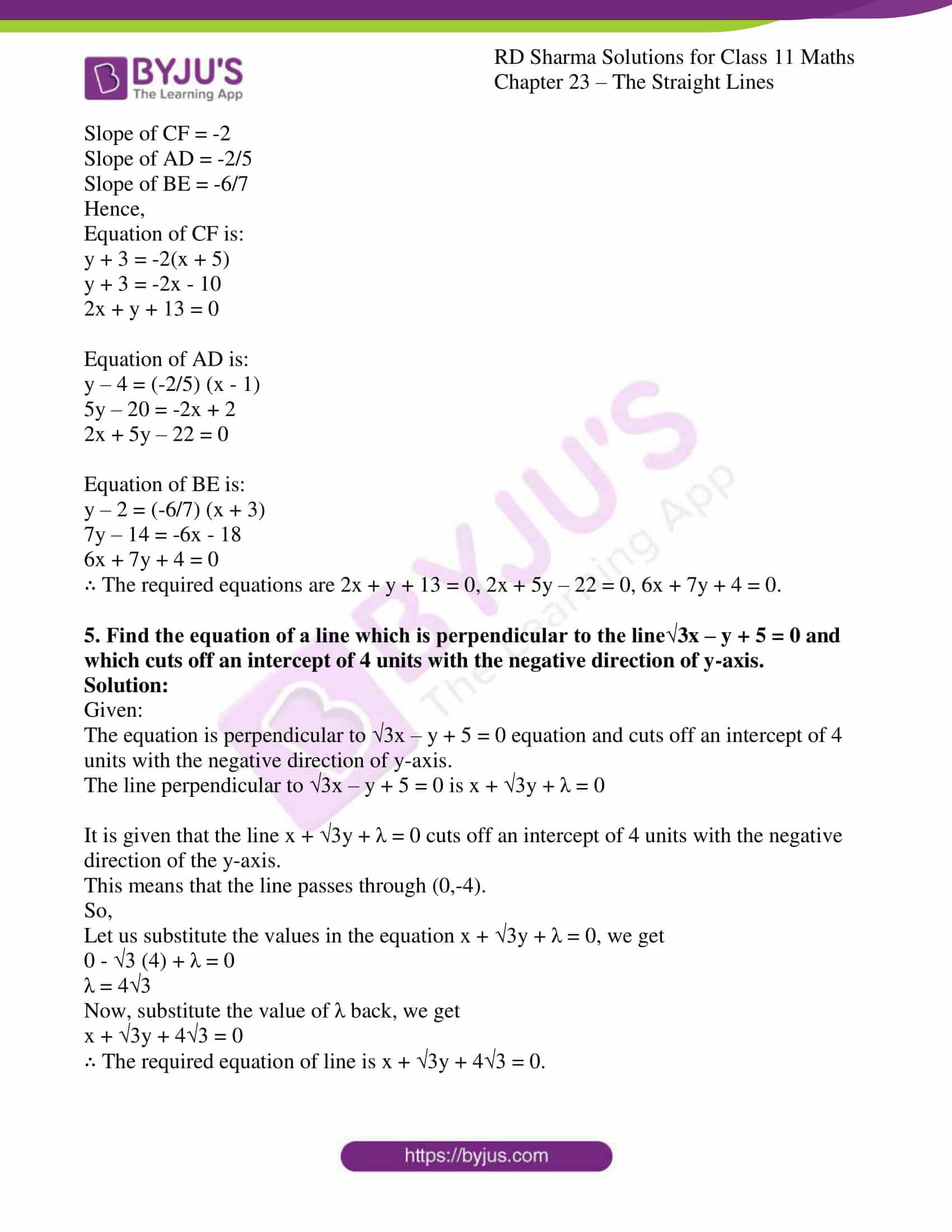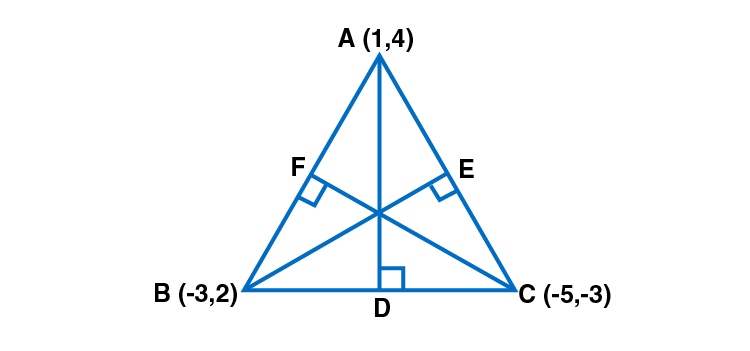# RD Sharma Solutions for Class 11 Chapter 23 - The Straight Lines Exercise 23.12

In this section, we shall deal with the concepts based on lines parallel and perpendicular to a given line, with examples, for students to gain knowledge about the concepts. Students who aim to secure high marks in their board exams are advised to practice the solutions on a daily basis by referring to RD Sharma Class 11 Solutions. Practising the textbook questions will help you in analyzing your level of preparation and knowledge of the concept. The pdf of RD Sharma Class 11 Maths Solutions can be easily downloaded, from the links provided below.

## Download the pdf of RD Sharma Solutions for Class 11 Maths Exercise 23.12 Chapter 23 – The Straight Lines### Access answers to RD Sharma Solutions for Class 11 Maths Exercise 23.12 Chapter 23 – The Straight Lines

#### EXERCISE 23.12 PAGE NO: 23.92

1. Find the equation of a line passing through the point (2, 3) and parallel to the line 3x– 4y + 5 = 0.

Solution:

Given:

The equation is parallel to 3x − 4y + 5 = 0 and pass through (2, 3)

The equation of the line parallel to 3x − 4y + 5 = 0 is

3x – 4y + λ = 0,

Where, λ is a constant.

It passes through (2, 3).

Substitute the values in above equation, we get

3 (2) – 4 (3) + λ = 0

6 – 12 + λ = 0

λ = 6

Now, substitute the value of λ = 6 in 3x – 4y + λ = 0, we get

3x − 4y + 6

∴ The required line is 3x − 4y + 6 = 0.

2. Find the equation of a line passing through (3, -2) and perpendicular to the line x – 3y + 5 = 0.

Solution:

Given:

The equation is perpendicular to x – 3y + 5 = 0 and passes through (3,-2)

The equation of the line perpendicular to x − 3y + 5 = 0 is

3x + y + λ = 0,

Where, λ is a constant.

It passes through (3, − 2).

Substitute the values in above equation, we get

3 (3) + (-2) + λ = 0

9 – 2 + λ = 0

λ = – 7

Now, substitute the value of λ = − 7 in 3x + y + λ = 0, we get

3x + y – 7 = 0

∴ The required line is 3x + y – 7 = 0.

3. Find the equation of the perpendicular bisector of the line joining the points (1, 3) and (3, 1).

Solution:

Given:

A (1, 3) and B (3, 1) be the points joining the perpendicular bisector

Let C be the midpoint of AB.

So, coordinates of C = [(1+3)/2, (3+1)/2]

= (2, 2)

Slope of AB = [(1-3) / (3-1)]

= -1

Slope of the perpendicular bisector of AB = 1

Thus, the equation of the perpendicular bisector of AB is given as,

y – 2 = 1(x – 2)

y = x

x – y = 0

∴ The required equation is y = x.

4. Find the equations of the altitudes of a ΔABC whose vertices are A (1, 4), B (-3, 2) and C (-5, -3).

Solution:

Given:

The vertices of ∆ABC are A (1, 4), B (− 3, 2) and C (− 5, − 3).

Now let us find the slopes of ∆ABC.Slope of AB = [(2 – 4) / (-3-1)]

= ½

Slope of BC = [(-3 – 2) / (-5+3)]

= 5/2

Slope of CA = [(4 + 3) / (1 + 5)]

= 7/6

Thus, we have:

Slope of CF = -2

Slope of BE = -6/7

Hence,

Equation of CF is:

y + 3 = -2(x + 5)

y + 3 = -2x – 10

2x + y + 13 = 0

y – 4 = (-2/5) (x – 1)

5y – 20 = -2x + 2

2x + 5y – 22 = 0

Equation of BE is:

y – 2 = (-6/7) (x + 3)

7y – 14 = -6x – 18

6x + 7y + 4 = 0

∴ The required equations are 2x + y + 13 = 0, 2x + 5y – 22 = 0, 6x + 7y + 4 = 0.

5. Find the equation of a line which is perpendicular to the line√3x – y + 5 = 0 and which cuts off an intercept of 4 units with the negative direction of y-axis.

Solution:

Given:

The equation is perpendicular to √3x – y + 5 = 0 equation and cuts off an intercept of 4 units with the negative direction of y-axis.

The line perpendicular to √3x – y + 5 = 0 is x + √3y + λ = 0

It is given that the line x + √3y + λ = 0 cuts off an intercept of 4 units with the negative direction of the y-axis.

This means that the line passes through (0,-4).

So,

Let us substitute the values in the equation x + √3y + λ = 0, we get

0 – √3 (4) + λ = 0

λ = 4√3

Now, substitute the value of λ back, we get

x + √3y + 4√3 = 0

∴ The required equation of line is x + √3y + 4√3 = 0.